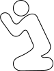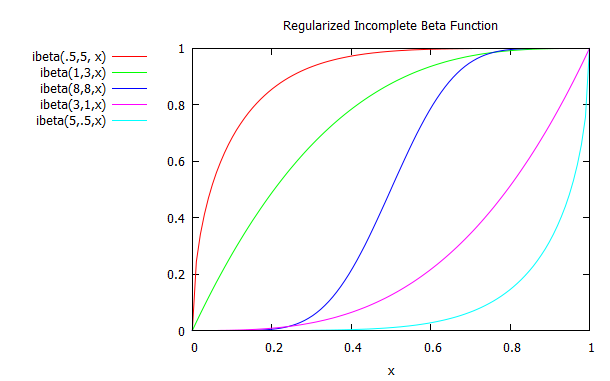CodePlea
Random thoughts on programming
06 Jan 2017

# Incomplete Beta Function in C

The Incomplete Beta function, or regularized incomplete beta function, seems to be one of those functions that should be built into the standard libraries, but is conspicuously missing. It shows up all over the place in statistics. It's crucial to Student's t distribution, which is likely the second most important distribution in statistics after the Gaussian distribution.I couldn't find an open-source implementation to fit my exact needs, so I'm going to give simple C code to compute it.

If you're just looking for code, check out the Github repo. If you want to learn how it works, keep reading.

## Why I Did Not Use Somebody Else's Code

Several open-source implementations of the incomplete beta function already exist, but none of them were going to work for my project.

The GNU Scientific Library has it, but it's a large dependency that you may not want to pull in for a single function. The incomplete beta function itself is somewhat intertwined with the library, and may not be easy to extract and use separately. It's GPL licensed.

The Cephes math library also has an incomplete beta function implementation, but it's a large function and the licensing is very uncertain and questionable.

The book Numerical Recipes has a very nice and short Incomplete Beta Function, but its licensing basically makes it unuseable. Despite that, I do recommend the book as a nice reference.

After looking around, I couldn't find a small, simple, and easy to use incomplete beta function in C with a liberal license. That's why I wrote my own from scratch.

## The Basics

Our starting point is the continued fraction representation, taken from the Digital Library of Mathematical Functions here.

It looks like this:

$$I_x(a,b)=\frac{x^a(1-x)^b}{aB(a,b)}\frac{1}{1+\frac{d_1}{1+\frac{d_2}{1+\frac{d_3}{1+...}}}}$$

The beta function is easily calculated from the gamma function as:

$$B(x,y) = \frac{\Gamma(x)\Gamma(y)}{\Gamma(x+y)}$$

Luckily, C99 gives us a log-gamma function, lgamma, which we can use. Keep in mind that adding logs is the same as multiplying. This works much better for us, because if we actually computed the gamma function itself it would quickly overflow. If you don't have a C99 compiler, then you'll need to implement log-gamma yourself.

Finally, we define the d terms in the first equation for the odd and even parts separately:

$$d_{2m+1}=-\frac{(a+m)(a+b+m)x}{(a+2m)(a+2m+1)}$$
$$d_{2m}=\frac{m(b-m)x}{(a+2m-1)(a+2m)}$$

This continued fraction rapidly converges when:

$$x \lt \frac{a+1}{a+b+2}$$

Lucky for us, the incomplete beta function has the following symmetry so we can always ensure fast convergence:

$$I_x(a,b) = 1 - I_{1-x}(b,a)$$

You can refer to the graph above to see the symmetry visually.

## Solving a Continued Fraction

Solving a continued fraction isn't exactly straightforward. You could, of course, just pick a point on the right and start solving right-to-left. The problem, however, is that you don't know where to start. You'd just be guessing and you have no way to refine your answer without starting all over.

I'm going to use Lentz's Algorithm to transform the continued fraction into something we can actually solve.

Lentz's Algorithm lets us calculate:

$$F = 1+\frac{a_1}{1+\frac{a_2}{1+\frac{a_3}{1+\frac{a_4}{1+...}}}}$$

As:

\begin{align*} D_0 &= 0 \\ C_0 &= 1 \\ F_0 &= 1 \\ D_i &= \frac{1}{1 + a_j D_{i-1}} \\ C_i &= 1 + \frac{a_j}{C_{i-1}} \\ F_i &= F_{i-1} C_i D_i \\ \end{align*}

It is useful to iterate until C*D becomes small.

Also note that you must ensure C and D never become zero (or you'll have a divide-by-zero issue). In practice, this is done by setting C or D to a very small value if either becomes too close to zero.

## My Implementation

With the above equations, I think coding up the incomplete beta function is fairly straight forward.

My goal was to make this as simple as possible, but still accurate and useful. Here is the code:

/*
* Copyright (c) 2016, 2017 Lewis Van Winkle
*/

#define TINY 1.0e-30

if (x < 0.0 || x > 1.0) return 1.0/0.0;

/*The continued fraction converges nicely for x < (a+1)/(a+b+2)*/
if (x > (a+1.0)/(a+b+2.0)) {
return (1.0-incbeta(b,a,1.0-x)); /*Use the fact that beta is symmetrical.*/
}

/*Find the first part before the continued fraction.*/
const double lbeta_ab = lgamma(a)+lgamma(b)-lgamma(a+b);
const double front = exp(log(x)*a+log(1.0-x)*b-lbeta_ab) / a;

/*Use Lentz's algorithm to evaluate the continued fraction.*/
double f = 1.0, c = 1.0, d = 0.0;

int i, m;
for (i = 0; i <= 200; ++i) {
m = i/2;

double numerator;
if (i == 0) {
numerator = 1.0; /*First numerator is 1.0.*/
} else if (i % 2 == 0) {
numerator = (m*(b-m)*x)/((a+2.0*m-1.0)*(a+2.0*m)); /*Even term.*/
} else {
numerator = -((a+m)*(a+b+m)*x)/((a+2.0*m)*(a+2.0*m+1)); /*Odd term.*/
}

/*Do an iteration of Lentz's algorithm.*/
d = 1.0 + numerator * d;
if (fabs(d) < TINY) d = TINY;
d = 1.0 / d;
c = 1.0 + numerator / c;
if (fabs(c) < TINY) c = TINY;

const double cd = c*d;
f *= cd;

/*Check for stop.*/
if (fabs(1.0-cd) < 1.0e-8) {
return front * (f-1.0);
}
}

return 1.0/0.0; /*Needed more loops, did not converge.*/

## Bonus - Student's t Cumulative Distribution Function

As I mentioned previously, the incomplete beta function is very useful when working with Student's t distribution. Here's code for the cumulative distribution function. It's useful for statistical test such as difference of means.

double student_t_cdf(double t, double v) {
/*The cumulative distribution function (CDF) for Student's t distribution*/
double x = (t + sqrt(t * t + v)) / (2.0 * sqrt(t * t + v));
double prob = incbeta(v/2.0, v/2.0, x);
return prob;
}